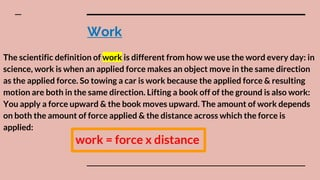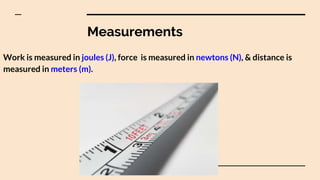Diese Präsentation wurde erfolgreich gemeldet.
Anzeige
Anzeige
Anzeige
Anzeige
Anzeige
Anzeige
Anzeige
Anzeige
Anzeige
Anzeige
Anzeige×

1 von 21 Anzeige

# Work & Machines

Teaches students about work & its measurements to physics, ranging from grades 6-8 grades. Also, teaches the difference between simple and compound machines, for example, inclined planes. Finally, teaches students how work can be understood as energy & efficiency.

Teaches students about work & its measurements to physics, ranging from grades 6-8 grades. Also, teaches the difference between simple and compound machines, for example, inclined planes. Finally, teaches students how work can be understood as energy & efficiency.

Anzeige
Anzeige

### Work & Machines

1. 1. Work & Machines By Emmanuel Dikolelay
2. 2. Work The scientific definition of work is different from how we use the word every day: in science, work is when an applied force makes an object move in the same direction as the applied force. So towing a car is work because the applied force & resulting motion are both in the same direction. Lifting a book off of the ground is also work: You apply a force upward & the book moves upward. The amount of work depends on both the amount of force applied & the distance across which the force is applied: work = force x distance
3. 3. Measurements Work is measured in joules (J), force is measured in newtons (N), & distance is measured in meters (m).
4. 4. Calculations To calculate work, we can only input force that is in the same direction of motion, meaning, if you are holding a basket of laundry, you won´t be doing work as you walk down a hall, but as soon as you walk up the stairs you are doing work. Why ? The force applied to the laundry basket to overcome gravity & pick it up is vertical; when you work down the hall, the motion of the basket is horizontal (not vertical), so you cannot include the horizontal distance in your calculation of work. When you walk up the stairs, the force is vertical (because you have to overcome gravity) & the motion is also vertical, so you are doing ¨work¨!
5. 5. Calculations (con.) Sometimes the force is only partially in the direction of motion. For example, if you can´t fully carry the trash, you lift up a little as you drag the bag (to overcome sliding friction), so you apply force in two directions: vertical & horizontal. However, because the bag is only moving across the floor, only the amount of force that is applied in the horizontal direction is considered ¨work¨.
6. 6. Power Power is the rate at which work is done, in other words, how quickly or slowly work is done. More powerful machines perform work faster. power = work time Power is measured with watts (W), & time is measured in seconds (s).
7. 7. Simple Machines To make work easier, humans invented machines. When you think of a machine, you might think of a tractor or a car, but a machine can be super simple. A machine is anything that makes work easier, even a ramp is a machine. A simple machine doesn´t reduce the total amount of work that is accomplished, but it decreases the amount of force required to do the same amount of work by increasing the distance.
8. 8. Simple Machines vs Compound Machines A simple machine is a machine that does work with a single motion, as opposed to a compound machine, which combines a number of simple machines to produce a more complicated machine, like a can opener. work: applying a force over a distance. The force must be in the same direction as movement J = N x M
9. 9. Inclined Plane An inclined plane or ramp is an example of a simple machine. It reduces the amount of effort required by increasing the work´s distance. Think about pushing a really heavy box into the back of the truck. With a ramp, you can roll the box up the ramp, which requires much less force than lifting the box into the truck. The box ends up at the same height, so the same amount of work is accomplished. However, because you pushed the box across a greater distance, less effort was required to raise the object the same distance. Egyptians built their pyramids using really long ramps.
10. 10. Wedge A wedge is a movable inclined plane, & it reduces the amount of work required to split or lift objects. Some examples of wedges are knives, axes, doorstops, & plows. To reduce the amount of effort required to cut firewood, people use wedge-shaped axes to split wood. It has to travel farther, but an axe requires less force than simply pulling a log in half with your bare hands.
11. 11. Screw A screw is a wedge (an inclined plane) wrapped around a shaft or post. As you turn the screw, the wedge pushes the object up the shaft (or the screw into the object). The amount of force required to secure a screw into the wall is less than it would be to hammer a nail of the same size. That´s because the spiral of the screw has to go to a much farther distance because it is turned so many times.
12. 12. Lever A lever reduces the amount of effort required to lift something. A lever is like a seesaw: a rigid bar or plank with a pivot point, called a fulcrum. When you apply a force, called effort, to one side of the fulcrum, the load on the other side also moves. Think about sitting on a seesaw with a friend, when you push down on the seat, your friend goes up into the air. Even if your friend is twice your mass, you can lift him by having him sit closer to the fulcrum. You push over more distance, so you need less force.
13. 13. Lever Classification on Force Levers are classified according to where the fulcrum & load are placed & where you apply the force, or effort:
14. 14. First Class The fulcrum is somewhere in the middle, & the load & effort are on each side of the fulcrum (like a seesaw).
15. 15. Second Class The fulcrum is to one side, the load is to the middle, & the effort is on the other side (like a wheelbarrow).
16. 16. Third Class The fulcrum is to one side, the effort is to the middle, & the load is on the other side (like your arm when you lift a weight).
17. 17. Wheel & Axle A wheel & axle make it easier to turn something by attaching a larger wheel to an axle, or rod, which is essentially a smaller wheel. There are two ways wheels & axles are used:
18. 18. They Increase the Output Force Turning a larger wheel requires less force than turning a smaller wheel (the larger wheel is moved a farther distance, so it requires less force for the same amount of work). Think about twisting open a faucet: It is much easier to turn a faucet handle a few inches than it would be to turn the pencil-thin neck. The axle exerts the output force.
19. 19. They Decrease the Distance Required to Move the Wheel Turning the smaller wheel requires more force than turning the larger wheel, but the smaller wheel must be turned a much smaller distance to accomplish the same work output. This mechanism is used on a bike: You apply the force over a shorter distance with the pedal, & the back wheel exerts less force over a longer distance. The wheel exerts the output force.
20. 20. Pulleys A pulley is a wheel with a rope wrapped around it. The rope fits into a groove on the wheel, & it is used to either magnify the force you apply to the rope (if you arrange a system of two or more pulleys) or change the direction of the force so it is easier to pull.
21. 21. Work as Energy & Efficiency The energy of an object increases as you do work on the object. For example, when you push an object, it begins to move, & that motion is a form of energy. Work is equivalent to energy, so all of the work done on an object is conserved in the form of energy. Energy comes in many forms, such as heat & motion. If you are doing work on an object & some of the energy you expend is lost in the form of heat (such as the heat the friction produces), you have lost some of your work. How much work, or energy, you lost to heat determines efficiency. A machine that doesn´t lose much energy to heat produces more work & is therefore more efficient.